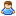1.You are currently browsing our forum as a guest. Create your own forum account to access all forum functionality.

# Can you list the raw materials required to build a MyProductionItem?

Discussion in 'Programming (In-game)' started by DreadedEntity, May 29, 2020.

This last post in this thread was made more than 31 days old.

Messages:
2
I'm hoping there is a way accessible through the API to get this. For example, making a steel plate requires 7 iron. I've started to hardcode each item, which isn't too bad since there's only 26, but if anything changed during a future update I'd have to also update my script, also there's the fact that (while sort of rare) some mods add new components to the game, which I have no knowledge of, so I'm also losing mod support.

Here's the script I'm working on, you can see in the bottom function where I've started the creation of a giant switch in which I hardcode every component in the game:
Code:
```public void Main(string argument, UpdateType updateSource)
{
List<IMyAssembler> blocks = new List<IMyAssembler>();
IMyBlockGroup group = GridTerminalSystem.GetBlockGroupWithName("testAssembler");
group.GetBlocksOfType<IMyAssembler>(blocks);
List<MyProductionItem> queueTemp = new List<MyProductionItem>();

List<MyProductionItem> masterQueue = new List<MyProductionItem>();

List<double> allMaterials = new List<double>(new double);
for (int i = 0; i < allMaterials.Count; i++) {
allMaterials[i] = 0;
}

for (int i = 0; i < blocks.Count; i++) {
blocks[i].GetQueue(queueTemp);
}
bool debug = true;
if (debug) {
for (int i = 0; i < masterQueue.Count; i++) {
Echo(masterQueue[i].ItemId.ToString());
Echo(masterQueue[i].Amount.RawValue.ToString());
Echo("" + (masterQueue[i].Amount.RawValue / 1000000));
}
} else {
for (int i = 0; i < masterQueue.Count; i++) {
List<double> temp = translateProductionItem(Convert.ToInt32(masterQueue[i].ItemId), Convert.ToInt32(masterQueue[i].Amount.RawValue / 1000000));
}

for (int i = 0; i < allMaterials.Count; i++){
Echo(allMaterials[i].ToString());
}
}
}

public List<double> addLists(List<double> l1, List<double> l2) {
for (int i = 0; i < l1.Count; i++) {
l1[i] += l2[i];
}
return l1;
}

public List<double> multiplyProduction(List<double> mats, int amount) {
for (int i = 0; i < mats.Count; i++) {
mats[i] *= amount;
}
return mats;
}

public List<double> translateProductionItem(int item, int amount) {
List<double> mats = new List<double>(new double);
switch (item) {
case 3372: {
mats = 4;
mats = 5/3;
mats = 1;
break;
}
default: {
break;
}
}

return multiplyProduction(mats, amount);
}
```